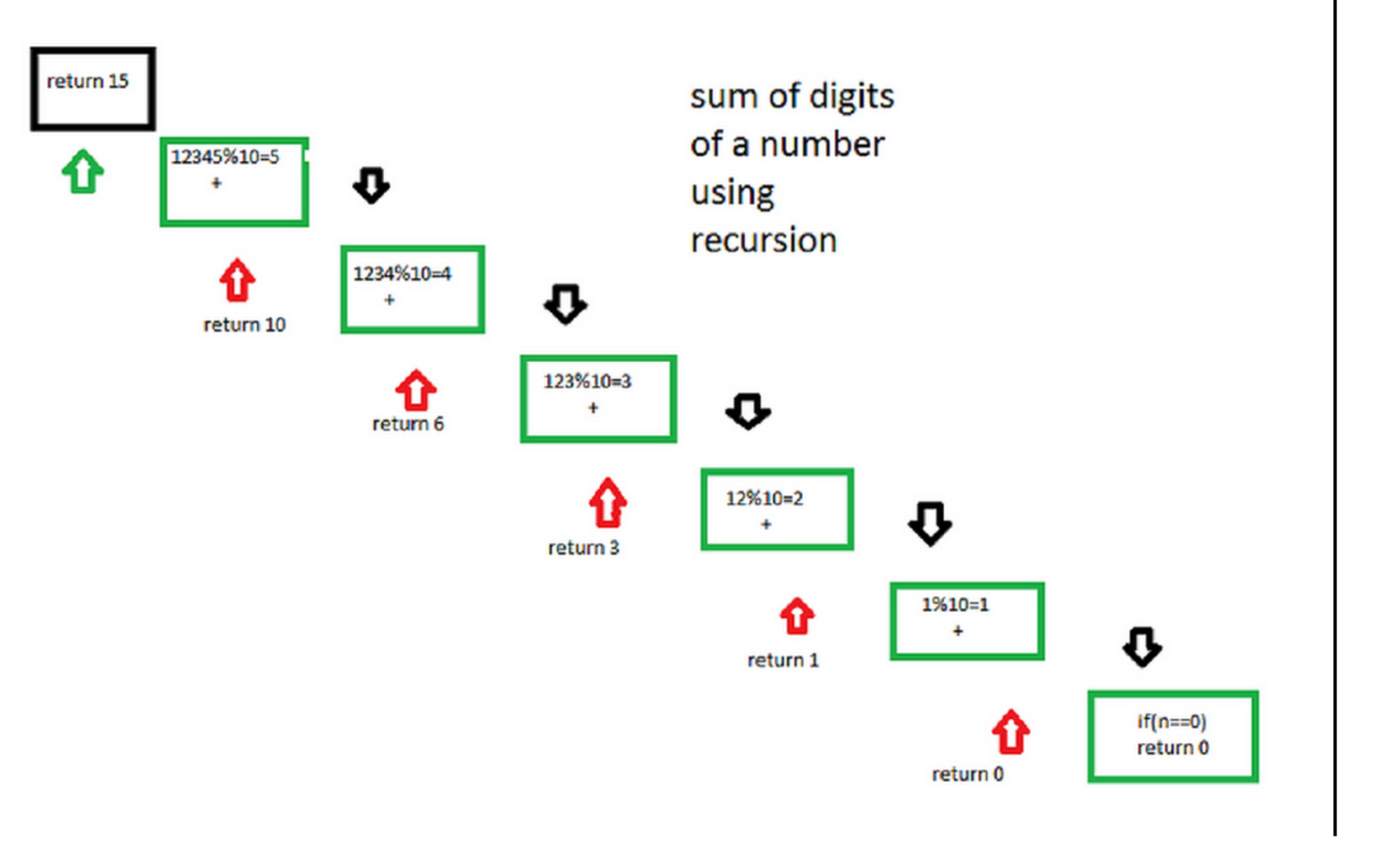# Sum of digit of a number using recursion

Given a number, we need to find sum of its digits using recursion.

Examples:

```Input : 12345
Output : 15

Input : 45632
Output :20
```

## Recommended: Please try your approach on {IDE} first, before moving on to the solution.

Step by step process for better understanding of how the algorithm works.
Let number be 12345.
Step 1-> 12345 % 10 which is equal-too 5 + ( send 12345/10 to next step )
Step 2-> 1234 % 10 which is equal-too 4 + ( send 1234/10 to next step )
Step 3-> 123 % 10 which is equal-too 3 + ( send 123/10 to next step )
Step 4-> 12 % 10 which is equal-too 2 + ( send 12/10 to next step )
Step 5-> 1 % 10 which is equal-too 1 + ( send 1/10 to next step )
Step 6-> 0 algorithm stops
following diagram will illustrate the process of recursion## C++

 `// Recursive C++ program to find sum of digits  ` `// of a number  ` `#include   ` `using` `namespace` `std;  ` ` `  `// Function to check sum of digit using recursion  ` `int` `sum_of_digit(``int` `n)  ` `{  ` `    ``if` `(n == 0)  ` `    ``return` `0;  ` `    ``return` `(n % 10 + sum_of_digit(n / 10));  ` `}  ` ` `  `// Driven code  ` `int` `main()  ` `{  ` `    ``int` `num = 12345;  ` `    ``int` `result = sum_of_digit(num);  ` `    ``cout << ``"Sum of digits in "``<< num  ` `       ``<<``" is "``<

## C

 `// Recursive C program to find sum of digits  ` `// of a number ` `#include ` ` `  `// Function to check sum of digit using recursion ` `int` `sum_of_digit(``int` `n) ` `{ ` `    ``if` `(n == 0) ` `       ``return` `0; ` `    ``return` `(n % 10 + sum_of_digit(n / 10)); ` `} ` ` `  `// Driven Program to check above ` `int` `main() ` `{ ` `    ``int` `num = 12345; ` `    ``int` `result = sum_of_digit(num); ` `    ``printf``(``"Sum of digits in %d is %d\n"``, num, result); ` `    ``return` `0; ` `} `

## Java

 `// Recursive java program to  ` `// find sum of digits of a number ` `import` `java.io.*; ` ` `  `class` `sum_of_digits ` `{ ` `    ``// Function to check sum  ` `    ``// of digit using recursion ` `    ``static` `int` `sum_of_digit(``int` `n) ` `    ``{  ` `        ``if` `(n == ``0``) ` `            ``return` `0``; ` `        ``return` `(n % ``10` `+ sum_of_digit(n / ``10``)); ` `    ``} ` ` `  `    ``// Driven Program to check above ` `    ``public` `static` `void` `main(String args[]) ` `    ``{ ` `        ``int` `num = ``12345``; ` `        ``int` `result = sum_of_digit(num); ` `        ``System.out.println(``"Sum of digits in "` `+  ` `                           ``num + ``" is "` `+ result); ` `    ``} ` `} ` ` `  `// This code is contributed by Anshika Goyal. `

## Python3

 `# Recursive Python3 program to  ` `# find sum of digits of a number ` ` `  `# Function to check sum of ` `# digit using recursion ` `def` `sum_of_digit( n ): ` `    ``if` `n ``=``=` `0``: ` `        ``return` `0` `    ``return` `(n ``%` `10` `+` `sum_of_digit(``int``(n ``/` `10``))) ` ` `  `# Driven code to check above ` `num ``=` `12345` `result ``=` `sum_of_digit(num) ` `print``(``"Sum of digits in"``,num,``"is"``, result) ` ` `  `# This code is contributed by "Sharad_Bhardwaj". `

## C#

 `// Recursive C# program to  ` `// find sum of digits of a number ` `using` `System; ` ` `  `class` `GFG { ` `     `  `    ``// Function to check sum  ` `    ``// of digit using recursion ` `    ``static` `int` `sum_of_digit(``int` `n) ` `    ``{  ` `        ``if` `(n == 0) ` `            ``return` `0; ` `             `  `        ``return` `(n % 10 + sum_of_digit(n / 10)); ` `    ``} ` ` `  `    ``// Driven Program to check above ` `    ``public` `static` `void` `Main() ` `    ``{ ` `        ``int` `num = 12345; ` `        ``int` `result = sum_of_digit(num); ` `        ``Console.WriteLine(``"Sum of digits in "` `+  ` `                           ``num + ``" is "` `+ result); ` `    ``} ` `} ` ` `  `// This code is contributed by Anant Agarwal. `

## PHP

 ` `

Output:

```Sum of digits in 12345 is 15
```

Attention reader! Don’t stop learning now. Get hold of all the important DSA concepts with the DSA Self Paced Course at a student-friendly price and become industry ready.

My Personal Notes arrow_drop_upCheck out this Author's contributed articles.

If you like GeeksforGeeks and would like to contribute, you can also write an article using contribute.geeksforgeeks.org or mail your article to contribute@geeksforgeeks.org. See your article appearing on the GeeksforGeeks main page and help other Geeks.

Please Improve this article if you find anything incorrect by clicking on the "Improve Article" button below.

Improved By : jit_t, SHUBHAMSINGH10

Article Tags :
Practice Tags :

3

Please write to us at contribute@geeksforgeeks.org to report any issue with the above content.